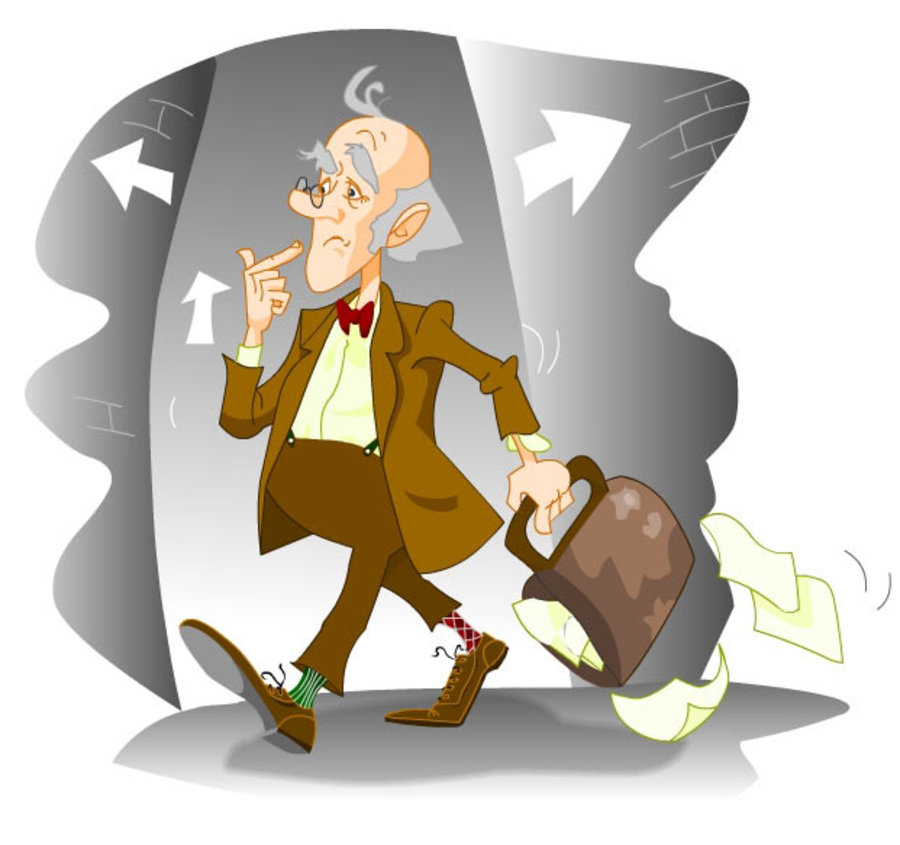# Professor's probability!

Probability Level 4An absent minded professor lost his latest set of question papers. In a panic, he decided to give his students marks chosen from a uniform distribution of the reals from 0 to 100.

But there is a problem. Two of the students in his class are identical twins, who study together and so get the similar marks. If their marks differ by more than $\large 20$, they will suspect that something is wrong.

The probability that the professor does not attract suspicion can be expressed in the form $\large \frac{a}{b}$, where $\large a$ and $\large b$ are positive coprime integers, determine the value of $\large a+b$.

Details and Assumptions
The marks need not be integers, they are simply real numbers. The maximum marks in the test were $\large 100$, and minimum $\large 0$.

×